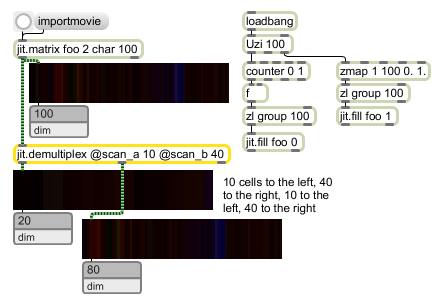# jit.demultiplex

Demultiplex (deinterleave) a single matrix into two matrices

## Description

The jit.demultiplex object takes a single matrix as input, and derives two output matrices from it by demultiplexing across a specified dimension. Specifically, the object takes a set of scanlines across the input matrix, placing these into the first output matrix, and then takes the next set of scanlines, placing those into the second output matrix, alternating between the two until the input matrix's data is exhausted.

## Matrix Operator

matrix inputs:1, matrix outputs:2
 Name IOProc Planelink Typelink Dimlink Plane Dim Type out n/a 0 0 0 1 1 char long float32 float64 out2 n/a 0 0 0 1 1 char long float32 float64

## Attributes

Name Type g/s Description
autoclear int Matrix clear following output flag (default = 1)
demultiplexdim int The dimension across which demultiplexing occurs (default = 0)
scan_a int The number of scanlines across the input matrix sampled into the first output matrix (default = 1)
To perform a standard deinterleave of NTSC video, scan_a and scan_b would both be set to 1.
scan_b int The number of scanlines across the input matrix sampled into the second output matrix (default = 1)
To perform a standard deinterleave of NTSC video, scan_a and scan_b would both be set to 1.

## Examples